Warning

# Registration

The assignment deals with image registration based on similarity transformations. The goal of the assignment is to reconstruct RGB images from the well-known Prokudin-Gorsky's collection of glass plate images. The collection contains triples of grayscale images showing the same scene. Each image in the triple was taken with a different color filter (red, green or blue) and thus it corresponds to one of the RGB channels. The images corresponding to individual channels need to be aligned before they can be composed. The alignment can be done automatically by registration.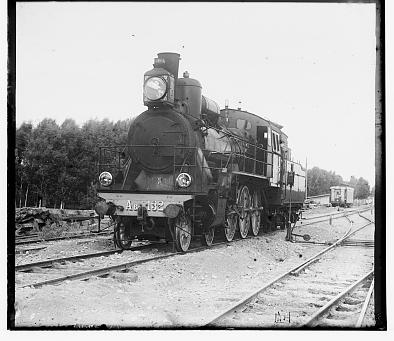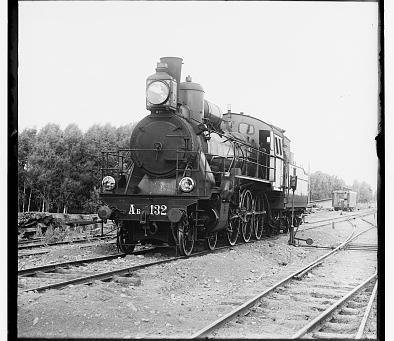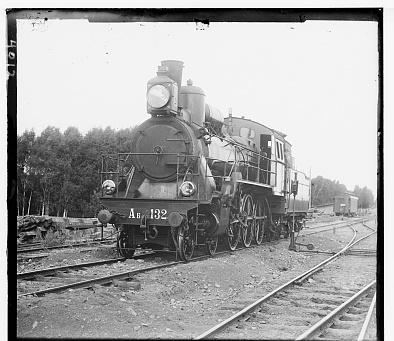Photograph taken with red filter Photograph taken with green filter Photograph taken with blue filter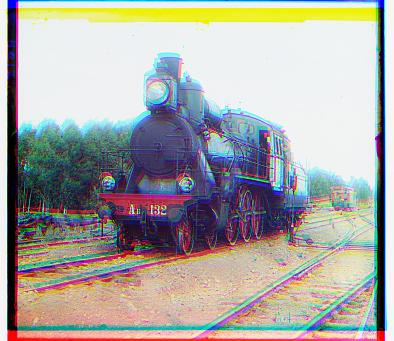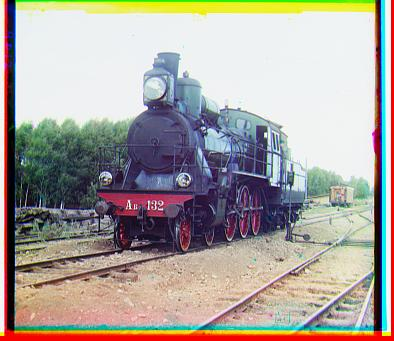Unaligned RGB channels (just overlaid) RGB channels aligned by registration

## Transformations

#### Geometry transformations

Coordinates of image pixels form a regular grid in 2D plane $\mathbb{R}^2$. Let us denote the coordinates of a pixel as $[x, y]$. The plane can be transformed by a certain transformation $T: \mathbb{R}^2 \rightarrow \mathbb{R}^2$ that assigns new coordinates $[x', y']$ to each pixel: $$T([x, y]) = [x', y']$$

In our case, we limit the considered transformation $T$ to a combination of rotation, scaling and translation. Therefore, $T$ is similarity transformation. Every similarity transformation can be written in the following form (with properly chosen coefficients $a_i, b_i$): \begin{align*} x' &= a_1 x + a_2 y + a_0\\ y' &= b_1 x + b_2 y + b_0 \end{align*}

Using the homogeneous coordinates, the transformation can be written as matrix multiplication. Refer the lecture slides for more details. $$\left[\begin{array}{c}x'\\y'\\1\end{array}\right] = \left[\begin{array}{c}a_1&a_2&a_0\\b_1&b_2&b_0\\0&0&1\\\end{array}\right] \left[\begin{array}{c}x\\y\\1\end{array}\right]$$

The more complex transformations can be composed from simple transformations. The composition is achieved by multiplying the matrices corresponding to simple transformations. We will need the following three simple transformations:

• Translation by the vector $[t_x, t_y]^T$. The corresponding translation matrix is: \begin{equation}M_{\mathrm{trans}}(t_x, t_y) = \left[\begin{array}{c}1&0&t_x\\0&1&t_y\\0&0&1\\\end{array}\right]\end{equation}
• Rotation by angle $\phi$ around the origin $[0, 0]^T$ in counter-clockwise direction. Note that the position of minus sign at $\sin\phi$ is flipped compared to the standard formulation. This is caused by the fact that y axis of the image coordinate system is reversed compared to the geometrical y axis. \begin{equation}M_{\mathrm{rot}}(\phi) = \left[\begin{array}{c}\cos\phi&\sin\phi&0\\-\sin\phi&\cos\phi&0\\0&0&1\\\end{array}\right]\end{equation}
• Scaling both x and y coordinates by the same factor $s$: \begin{equation}M_{\mathrm{scale}}(s) = \left[\begin{array}{c}s&0&0\\0&s&0\\0&0&1\\\end{array}\right]\end{equation}

We are interested in combination of image rotation around its center, scaling its size, and translation. It can be achieved by translating the grid by $[-m_x, -m_y]^T$ so that its center $[m_x, m_y]^T$ is aligned with the origin $[0, 0]^T$, scaling the grid by the factor $s$, rotating it around the origin by angle $\phi$, translating it back by $[m_x, m_y]^T$ and finally translating it by $[t_x, t_y]^T$ as required.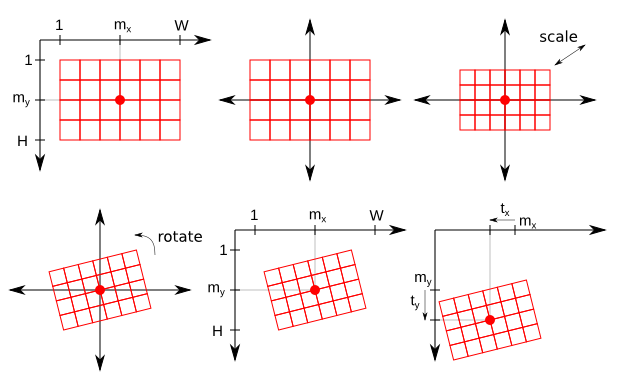The original coordinate grid is translated so that its center is aligned with the origin, scaled, rotated around the origin, translated back to its original position and translated as required.

#### Image transformations

If we want to apply certain transformation to the image $I$ to obtain the transformed image $I'$, we usually do not apply the geometry transformation directly. It would cause many problems including gaps in the transformed image or pixels mapped outside the image. Refer the lecture slides for further description.

We instead use the inverse transformation $T^{-1}$ satisfying: $$[x, y] = T^{-1}([x', y']) = T^{-1}(T([x, y]))$$

If we need to know the value $I'(x', y')$ in the pixel $[x', y']$ of the transformed image $I'$, we compute $[x, y]$ by applying $T^{-1}$ to $[x', y']$ and query the values of the source image $I$ in the vicinity of the point $[x, y]$. We usually employ linear or cubic interpolation, as described in the lecture slides.

#### Implementation

As we have already specified, we are interested in the similarity transformations comprehending image rotation around its center, scaling and translation. In Matlab, we will represent instances of such transformations by structures. The following structure represents translation by 1.5 pixels in the direction of x coordinate, 0.5 pixel in y coordinate, rotation of 10 degrees (not radians!) and scaling by multiplication factor 0.75:

t = struct('x', 1.5, 'y', -0.5, 'r', 10, 's', 0.75);

We will represent image pixels by 2D grids of x and y coordinates generated by Matlab function meshgrid. The following code will generate coordinates of a hypothetical image having width 10 and height 5:

[x', y'] = meshgrid(1:10, 1:5);

1. Explore the provided functions:
• t_inv = inverse_transformation(t): Return structure t_inv representing the transformation inverse to the transformation represented by the structure t.
• img_xy = sample_image(img, xt, yt): Interpolate the image img in the points specified by grids of coordinates x and y. The function is used to transform the image using the inverse transformation.
2. Implement the following function:
• [xt, yt] = transform_grid(x, y, t): Transform 2D grids of x and y coordinates by the specified transformation t, which is a combination of translation, rotation around the grid center and scaling.
3. Test your implementation using the script hw_registration_transform included in the package. We recommend you to test the proper functionality of simple transformations before combining them.

Based on the selected transformation, you should see one of the following outputs by running the testing script if you implement the required function correctly: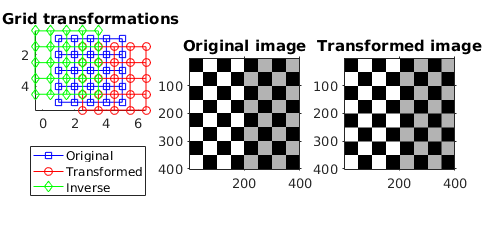Translation by 1.5 pixels in x and 0.5 pixels in y. Note that the transformed image is slightly translated.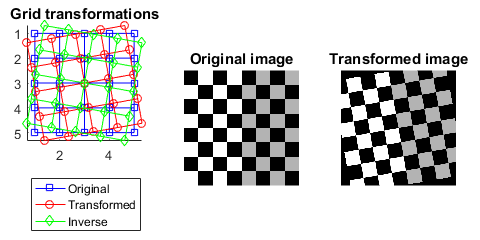Rotation by 10 degrees around the center.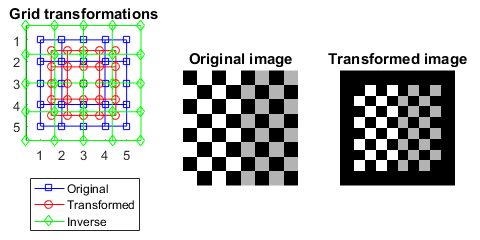Down-scaling by multiplication factor 0.75.

## Registration 1

Let suppose that we are given a small template image $I$ of size $H \times W$ and large reference image $I_\mathrm{ref}$. Let $T$ be a similarity transformation that transforms coordinate grid of the small template image to the reference image. The transformation $T$ determines $H \times W$ subimage $I_{\mathrm{ref}}^T$ of the reference image $I_\mathrm{ref}$: $$I_{\mathrm{ref}}^T(x, y) \approx I_{\mathrm{ref}}(T([x, y])) \text{ for } x = 1 \ldots W, y = 1 \ldots H$$

Note that the equality holds only approximately because the transformed coordinates $[x', y'] = T([x, y])$ are generally non-integer and the values of $I_{\mathrm{ref}}^T(x,y)$ are obtained by interpolation, as described previously. Given the template image $I \equiv$ img, the reference image $I_\mathrm{ref} \equiv$ img_ref and the transformation $T \equiv$ t, we can compute the transformed refence image $I_\mathrm{ref}^T \equiv$ img_ref_t with the following code:

[h, w] = size(img);   % size of the template image
[x, y] = meshgrid(1:w, 1:h);   % coodinate system of the template image
[xt, yt] = transform_grid(x, y, t);   % transformed coordinate system
img_ref_t = sample_image(img_ref, xt, yt);   % subimage of the reference image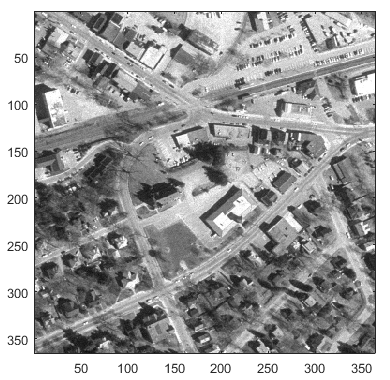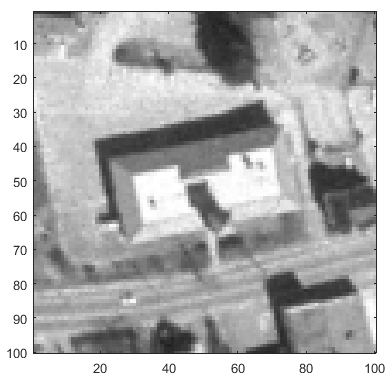Reference image $I_\mathrm{ref}$ Template image $I$ of size $100 \times 100$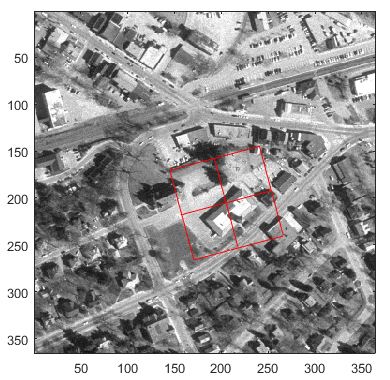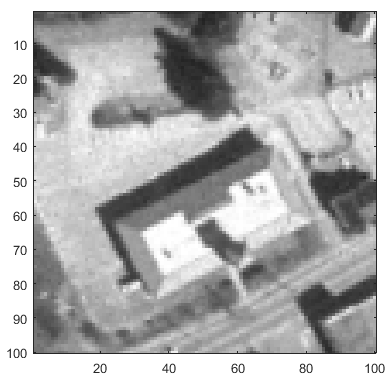Transformed $100 \times 100$ coordinate grid $100 \times 100$ subimage $I_\mathrm{ref}^T$ of the reference image

In the registration task, we explore certain space of possible transformations $\mathcal{T}$. Our goal is to find the optimum transformation $T^* \in \mathcal{T}$ such that the template image matches the subimage of the reference image. This can be formulated as an optimization task with respect to a certain cost function $f$ that evaluates differences of two equally sized images: $$T^* = \mathop{\text{argmin}}_{T\in{\mathcal{T}}} f(I, I_{\mathrm{ref}}^T)$$

There are many options for selecting the cost function $f$. Probably the simplest example is a sum of squared differences between values of individual pixels: $$f_\mathrm{SSD}(I_1, I_2) = \sum_{x = 1}^{W} \sum_{y = 1}^{H} ( I_1(x,y) - I_2(x,y) )^2$$

It remains to define the space of all considered transformations $\mathcal{T}$. In Matlab, we will use the following structure to describe range and step of each transformation parameter (x and y translation, rotation angle in degrees, scaling factor):

t_rng = struct('x_low', 10, 'x_high', 20, 'x_step', 1, ...
'y_low', -5, 'y_high', 15, 'y_step', 2, ...

The space $\mathcal{T}$ is given by the Cartesian product of all parameter values from the ranges. We search the space exhaustively. We generate all the possible transformations and select the one optimizing the cost function $f_\mathrm{SSD}$.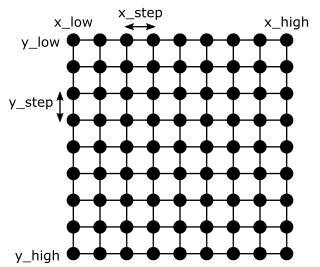Exhaustive search of all combinations of x and y translations from the specified ranges. Rotations and scaling factors add 3rd and 4th dimension to the searched grid.
1. Explore the provided function:
• t_space = transformation_space_exhaustive(t_rng): Generate space of transformations as the Cartesian product of all parameters from the specified ranges.
2. Implement the following functions:
• ssd = sum_of_squared_differences(img1, img2): Compute sum of squared differences between pixels of two images having the same size.
• t_optim = estimate_transformation_exhaustive(img, img_ref, cost_func, t_rng): Find the optimal transformation of the template image to the reference image by searching the space of all possible transformations exhaustively.
3. Test your implementation using the script hw_registration_image included in the package.

## Registration 2

We will now advance to registration of RGB channels from Prokudin-Gorsky's collection. The task can be solved as follows. The images corresponding to individual RGB channels are cropped at first to remove the black margins that could confuse the registration. One of the channels is selected as the reference one, i.e. it is considered to be $I_\mathrm{ref}$. Each of two remaining channels is then registered to the reference channel separately. In other words, one of the remaing channels is considered to be $I$ and the optimum transformation $T^*$ is found as before: $$T^* = \mathop{\text{argmin}}_{T\in{\mathcal{T}}} f(I, I_{\mathrm{ref}}^T)$$

The estimated transform $T^*$ is then applied to $I$ to align it with the reference channel $I_\mathrm{ref}$. The process is then repeated for the second unregistered channel. Finally, the reference channel and both aligned channels are composed to single RGB image.

### Hierarchical coarse to fine registration

The number of explored transformations can be reduced significantly by searching the space $\mathcal{T}$ hierarchically in coarse to fine manner. At the coarser level, a larger step is chosen for each transformation parameter and the optimum transformation is found over the coarse search space. At the finer level, only the neighborhood of the optimum parameter values from the coarser level is explored with a reduced step size. The following figure summarizes the described idea.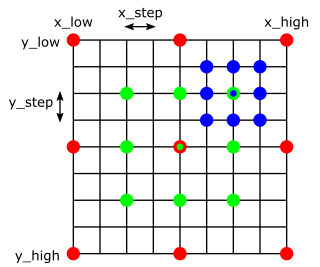Coarse to fine search of x and y translations on 3 levels of hierarchy. On the coarsest level 3, only the red combinations of parameters are explored, finding the optimum in the middle. On level 2, the optimum is found in the top-right combination. The finest level 1.

It is convenient to use a smoothed and down-scaled images on the coarser levels of the hierarchy. The cost function is evaluated faster on the down-scaled images. The smoothing propagates information to the neighboring pixels and reduces aliasing. You can get the idea from the following code. It is contained in the file estimate_transformation_hierarchical.m that you need to complete.

[h, w] = size(img);
t_optim = struct();

for level = num_levels:-1:1
% multiplicative factor for the current level
mult = 2^(level - 1);

% coordinate grid for the current level
x = linspace(1, w, ceil(w / mult));
y = % TODO
[x, y] = meshgrid(x, y);

% smooth both template and reference image
img_smooth = smooth_image(img, mult);
img_ref_smooth = % TODO

% subsample the smoothed template image in the grid coordinates
img_sampled = sample_image( % TODO

% generate space of transformations for the current level using the optimum
% transformation from the previous level
t_space = transformation_space_hierarchical(t_optim, level, num_levels, t_rng);

% find the optimum transformation in t_space and assign it to t_optim
t_optim = % TODO
end

The following figure shows run of the algorithm at single level of hierarchy. Both template image img and reference image img_ref are smoothed at first. The smoothed template image is then sampled by a regular grid whose step depends on the level. The grid is transformed for each transformation from t_space and used to sample the smoothed reference image img_ref_smooth. The cost of the transformation is evaluated on the sampled images.

The following figure shows the next loop of the algorithm at the finer level of hierarchy. Note that the images are less smoothed. The step of the sampling grid is reduced by half.

### Mutual information

Previously, we used sum of squared differences between corresponding pixels $f_\mathrm{SSD}(I_1, I_2)$ to evaluate similarity of two images $I_1$ and $I_2$. This cost function was suitable for searching a subimage in large reference image, because we were measuring differences of the same intensity functions. However, $f_\mathrm{SSD}$ is less suitable to compare intensities coming from various color channels. The red, green and blue values of single pixel are not equal in general, however, they are still statistically dependent.

We will use mutual information (MI) to measure the statistical dependence. We will evaluate the mutual information on the set of the corresponding intensity pairs: $$\big\{ [I_1(x,y), I_2(x,y)] \mid x = 1 \ldots W, y = 1 \ldots H \big\}$$

The intensity images $I_1$ and $I_2$ correspond to two different channels of single $H \times W$ RGB image in our case. The mutual information is computed as follows: \begin{align} \mathrm{MI} &\Big( \big\{ [I_1(x,y), I_2(x,y)] \mid x = 1 \ldots W, y = 1 \ldots H \big\} \Big)\\ =&\mathrm{Entropy} \Big( \big\{ I_1(x,y) \mid x = 1 \ldots W, y = 1 \ldots H \big\} \Big)\\ +&\mathrm{Entropy} \Big( \big\{ I_2(x,y) \mid x = 1 \ldots W, y = 1 \ldots H \big\} \Big)\\ -&\mathrm{Entropy} \Big( \big\{ [I_1(x,y), I_2(x,y)] \mid x = 1 \ldots W, y = 1 \ldots H \big\} \Big)\\ \end{align}

The entropies are computed based on histograms. Use quantization to 10 bins while computing histogram of intensities for single image, i.e. bin_centers = linspace(0, 1, 10). You can use the built-in function N = hist(Y, X) to compute the histogram. Use 10×10 bins for 2D histogram of intensity pairs from two images. You can use the built-in Matlab function N = hist3(X, CTRS) to compute the 2D histogram.

The histograms need to be normalized to form valid probabilistic distributions of intensities (or pairs of the corresponding intensities). The entropy of a discrete probabilistic distribution with probabilities $p_i$ where $i = 1 \ldots 10$ is given by the following formula: $$- \sum_{i = 1}^{10} p_i \log p_i$$

Here $\log$ is a natural logarithm. Note that $p_i = 0$ should be left out. The entropy of 2D distribution with probabilities $p_{i,j}$ is computed analogously.

The mutual information is higher when the corresponding intensities are statistically dependent and goes to zero for less dependent. Therefore, the negative value of the mutual information can be used as the cost function evaluating similarity of two registered RGB channels: $$f_\mathrm{MI}(I_1, I_2) = - \mathrm{MI} \Big( \big\{ [I_1(x,y), I_2(x,y)] \mid x = 1 \ldots W, y = 1 \ldots H \big\} \Big)$$

#### Implementation

1. Explore the provided functions:
• t_space = transformation_space_hierarchical(t, level, num_levels, t_rng): Generate space of transformations for one level of hieararchical estimator, using the optimum transformation from coarser level.
• img_smooth = smooth_image(img, sigma): Smooth the image by convolving it with Gaussian filter.
2. Finish implementation of the following function:
• t_optim = estimate_transformation_hierarchical(img, img_ref, cost_func, num_levels, t_rng): Find the optimum transformation by searching the transformation space hierarchically in coarse to fine manner.
3. Implement the following functions:
• mi = mutual_information(img1, img2): Compute mutual information of two intensity images using 10 bins.
• img = compose_rgb_image(rgb, t_rgb, ref_channel): Compose image from RGB channels and corresponding transformations.
4. Test your implementation using the script hw_registration_rgb included in the package. You can also use the previous script hw_registration_image for further testing.